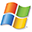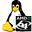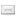ATOMS : Distfun details

# Distfun

Distribution functions
Details
Version
0.2
A more recent valid version with binaries for Scilab 5.3 exists: 0.4
Author
Michael Baudin
Owner Organization
INRIA
Maintainer
Michael Baudin
Category
Dependencies
Creation Date
March 24, 2012
Source created on
Scilab 5.3.x
Binaries available on
Scilab 5.3.x:Linux 32-bitWindows 32-bitWindows 64-bitmacOSLinux 64-bit
Install command
`--> atomsInstall("distfun")`
Report a bugDescription
```            The goal of this toolbox is to provide accurate distribution functions.
The provided functions are designed to be compatible with Matlab.

The goal of this toolbox is to provide accurate distribution functions.
The provided functions are designed to be compatible with Matlab.

Features
--------

Beta
* distfun_betacdf — Beta CDF
* distfun_betainv — Beta Inverse CDF
* distfun_betapdf — Beta PDF
* distfun_betarnd — Beta random numbers
* distfun_betastat — Beta mean and variance

Exponential
* distfun_expcdf — Exponential CDF
* distfun_expinv — Exponential Inverse CDF
* distfun_exppdf — Exponential PDF
* distfun_exprnd — Exponential random numbers
* distfun_expstat — Exponential mean and variance

Gamma
* distfun_gamcdf — Gamma CDF
* distfun_gaminv — Gamma Inverse CDF
* distfun_gampdf — Gamma PDF
* distfun_gamrnd — Gamma random numbers
* distfun_gamstat — Gamma mean and variance

LogNormal
* distfun_logncdf — Lognormal CDF
* distfun_logninv — Lognormal Inverse CDF
* distfun_lognpdf — Lognormal PDF
* distfun_lognrnd — Lognormal random numbers
* distfun_lognstat — LogNormal mean and variance

Normal
* distfun_normcdf — Normal CDF
* distfun_norminv — Normal Inverse CDF
* distfun_normpdf — Normal PDF
* distfun_normrnd — Normal random numbers
* distfun_normstat — Normal mean and variance

Uniform
* distfun_unifcdf — Uniform CDF
* distfun_unifinv — Uniform Inverse CDF
* distfun_unifpdf — Uniform PDF
* distfun_unifrnd — Uniform random numbers
* distfun_unifstat — Uniform mean and variance

Support
* distfun_erfcinv — Inverse erfc function
* distfun_getpath — Returns path of current module
```Files (6)Source code archive
```
```Linux 32-bit binary for Scilab 5.3.x
```Linux 32-bit
Automatically generated by the ATOMS compilation chain

```Windows 32-bit binary for Scilab 5.3.x
```Windows 32-bit
Automatically generated by the ATOMS compilation chain

```Windows 64-bit binary for Scilab 5.3.x
```Windows 64-bit
Automatically generated by the ATOMS compilation chain

```macOS binary for Scilab 5.3.x
```MacOSX version
Automatically generated by the ATOMS compilation chain

```Linux 64-bit binary for Scilab 5.3.x
```Linux 64-bit
Automatically generated by the ATOMS compilation chain

```News (0)Comments (1)Comment from Michael Baudin-- May 16, 2012, 02:18:48 PM
```Here are the changes in the v0.2.

distfun (0.2)
* Splitted grand into separate distfun_grand* gateways.